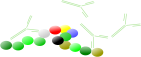### SPR-Pagesback
Data fitting
back
back

# Theory

The goal of data fitting is to fit a line through a data set. The computer program finds the best-fit values of the variables in the model (rate constants, affinities, receptor number), which can interpreted scientifically. Choosing a mathematical model is a scientific decision. You should base your choice on your understanding of chemistry or physiology. For example, the law of mass action explains many kinds of binding data. This section will help you to choose the model that describes your interaction.

## Kinetic models

This section will discuss how to choose a fitting model. Furthermore, it reviews the parameters commonly used in the models. The models are explained and the formulas are given. It will highlight the situation when you can use this model and when not. In addition, there is an example curve with kinetic values to study.

## Validation

After fitting a model to the curves a validation of the fitting and calculated parameter values must be done, even when the residuals are low! Inspect the residual plot for non-random distribution. Check the obtained parameters and asses if they are relevant. Is the association rate constant possible? Is the calculated response within the expected values?